## Friday, April 13, 2018

### Some new features to modernize `plotTree.wBars`

After posting a recent hack demonstrating how to plot stacked bars on a fan tree using `plotTree.wBars` in phytools I decided it might be useful to modernize the function a bit.

One feature I though I could add was dotted linking lines leading from the tip of the tree to either the tip label or the bar for non-ulrametric trees. The reason for this is because it can be very difficult to line-up the bar with its corresponding tip if the some tips terminate before the present.

Another feature that I thought I'd get rid of at the same time is the fact that for all x > 0 the behavior of the function is to attach the bars directly to the tip - regardless of whether or not it terminates in the present.

Here's what I mean:

``````library(phytools)
tree<-pbtree(n=100,b=1,d=0.5)
x<-fastBM(tree,bounds=c(0,Inf))
plotTree.wBars(tree,x,lwd=1)
``````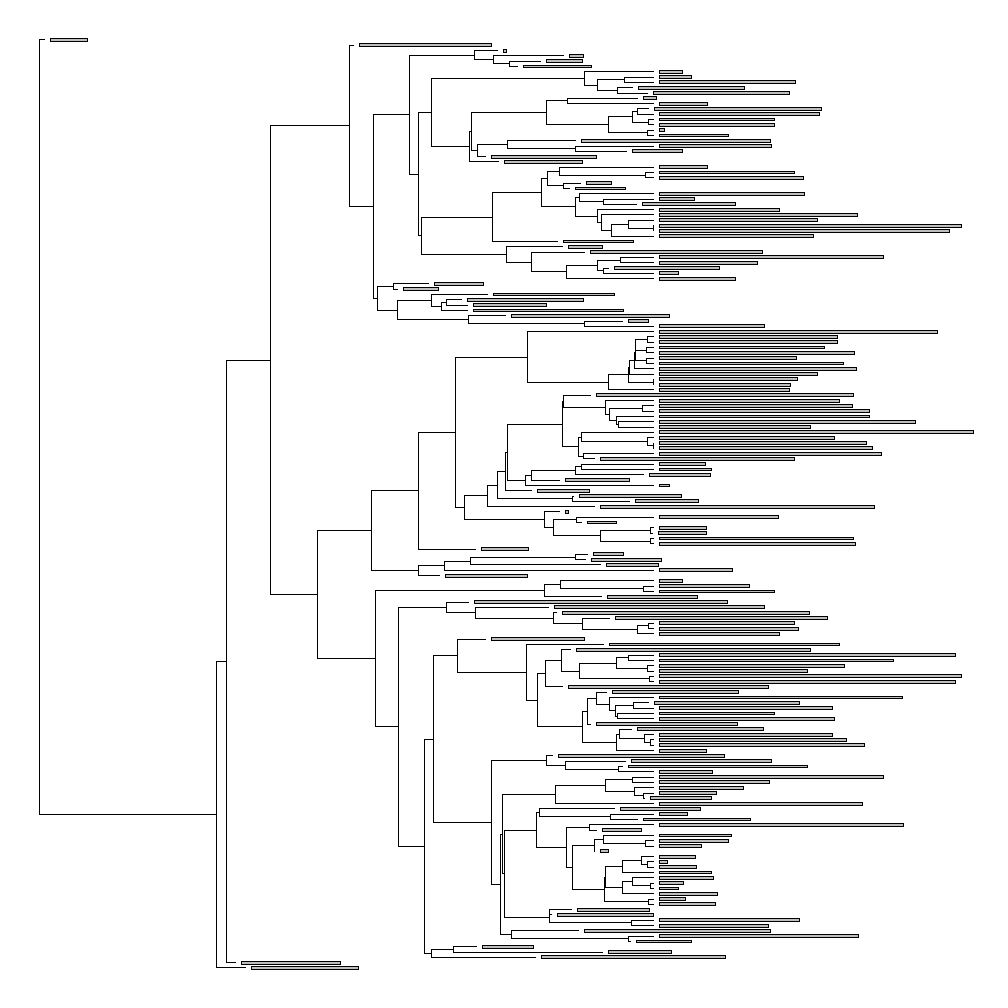or:

``````plotTree.wBars(tree,x,lwd=1,type="fan")
``````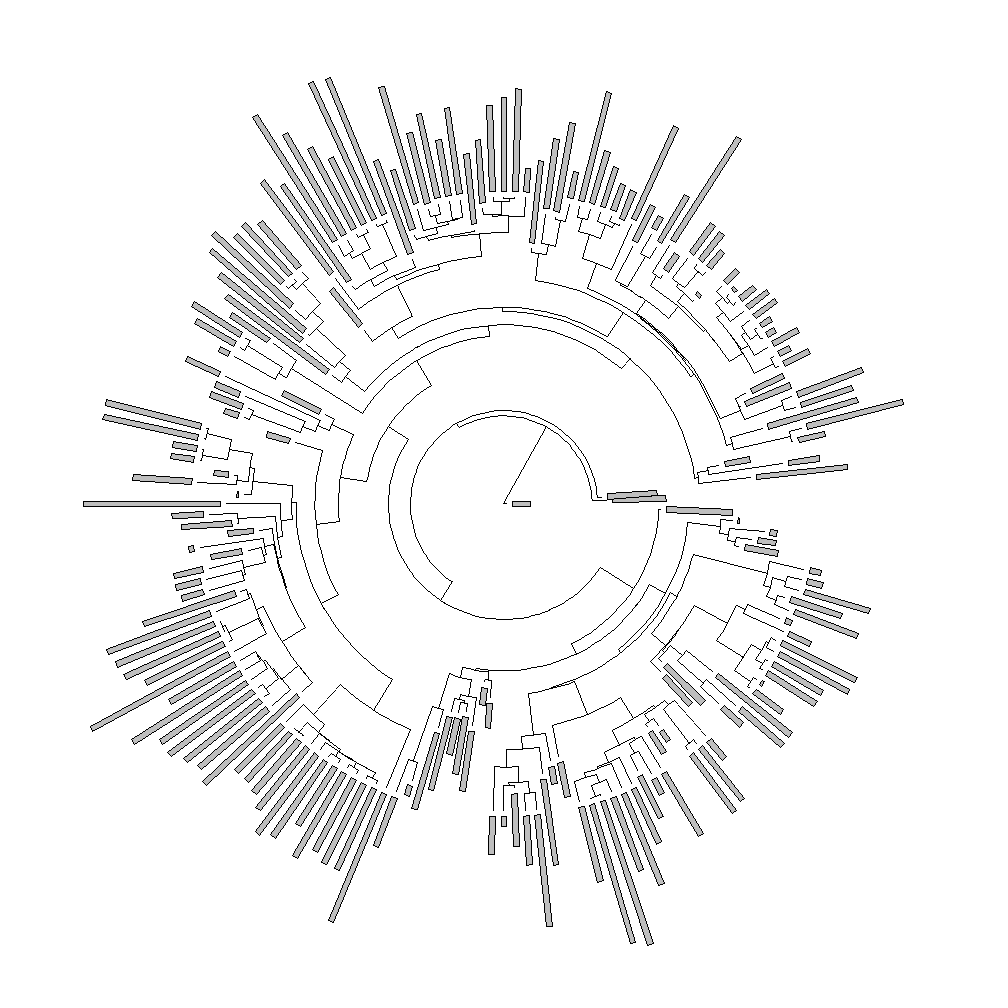Obviously this could be undesirable under some circumstances.

Note that the function does not behave this way when some or all values of x are negative:

``````y<-fastBM(tree)
plotTree.wBars(tree,y,lwd=1,type="fan")
``````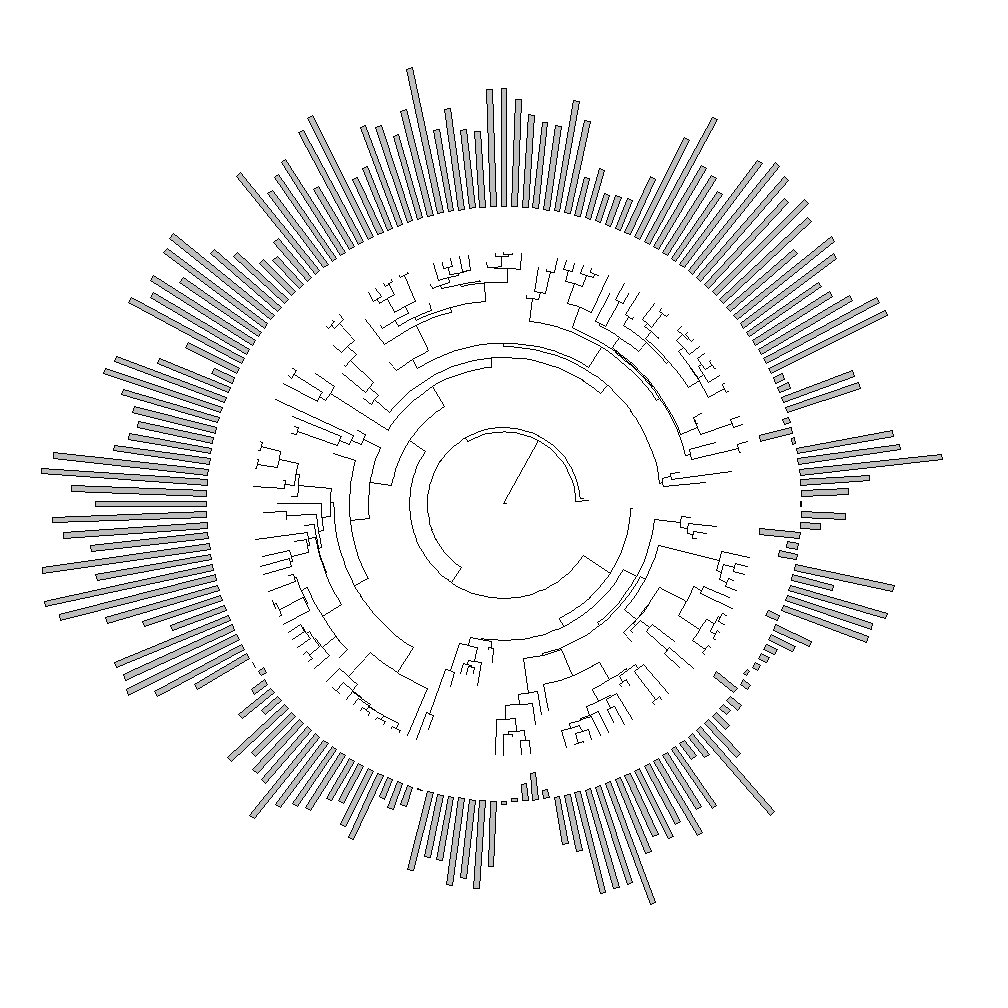Let's load the update version from GitHub & see how it compares. Note that we would normally just update phytools from GitHub using devtools.

``````source("https://raw.githubusercontent.com/liamrevell/phytools/master/R/plotTree.wBars.R")
plotTree.wBars(tree,x,lwd=1)
``````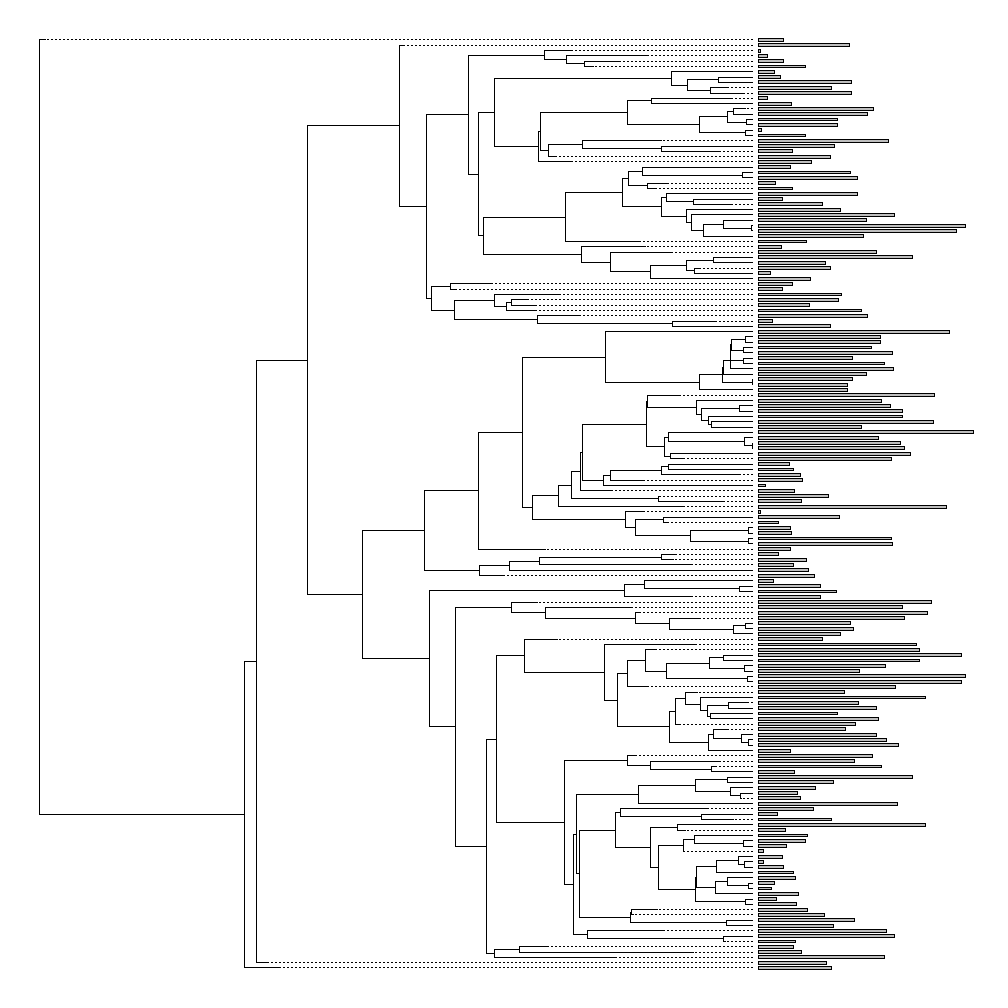``````## or
plotTree.wBars(tree,x,type="fan")
``````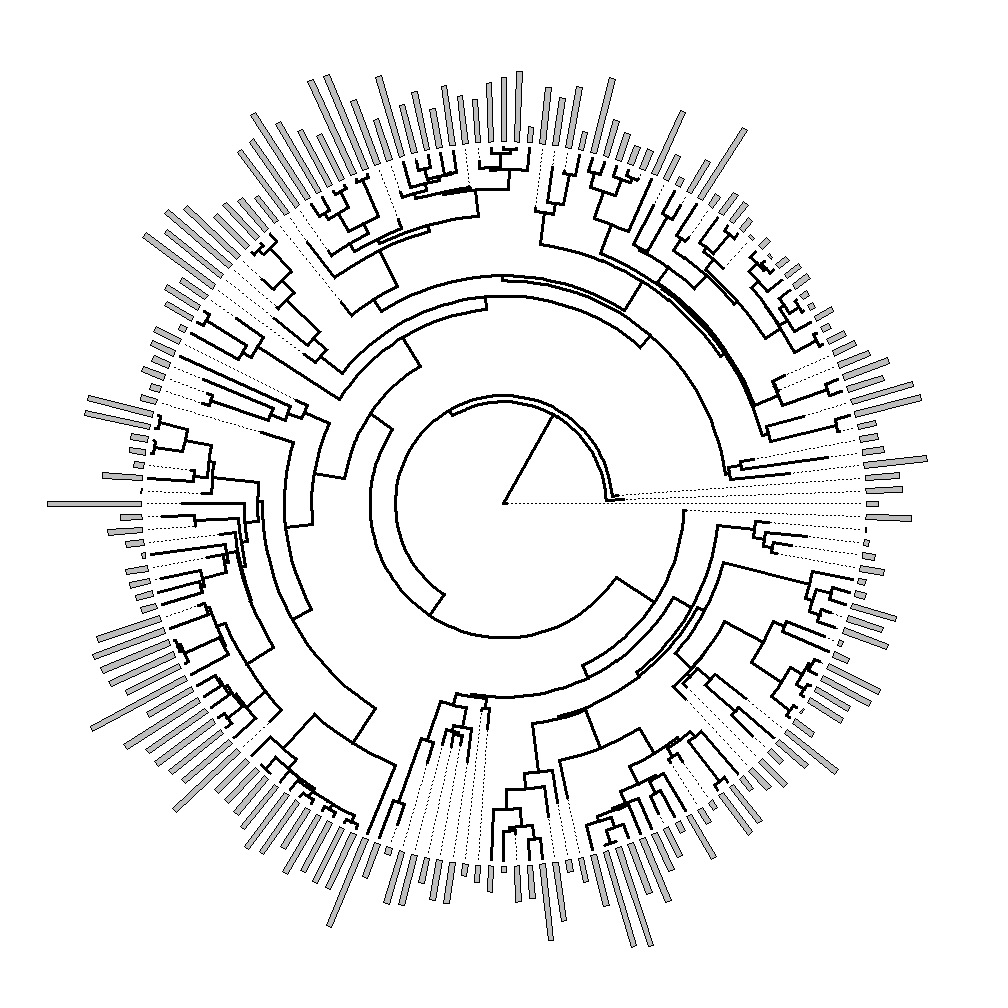``````## and
plotTree.wBars(tree,y,type="fan")
``````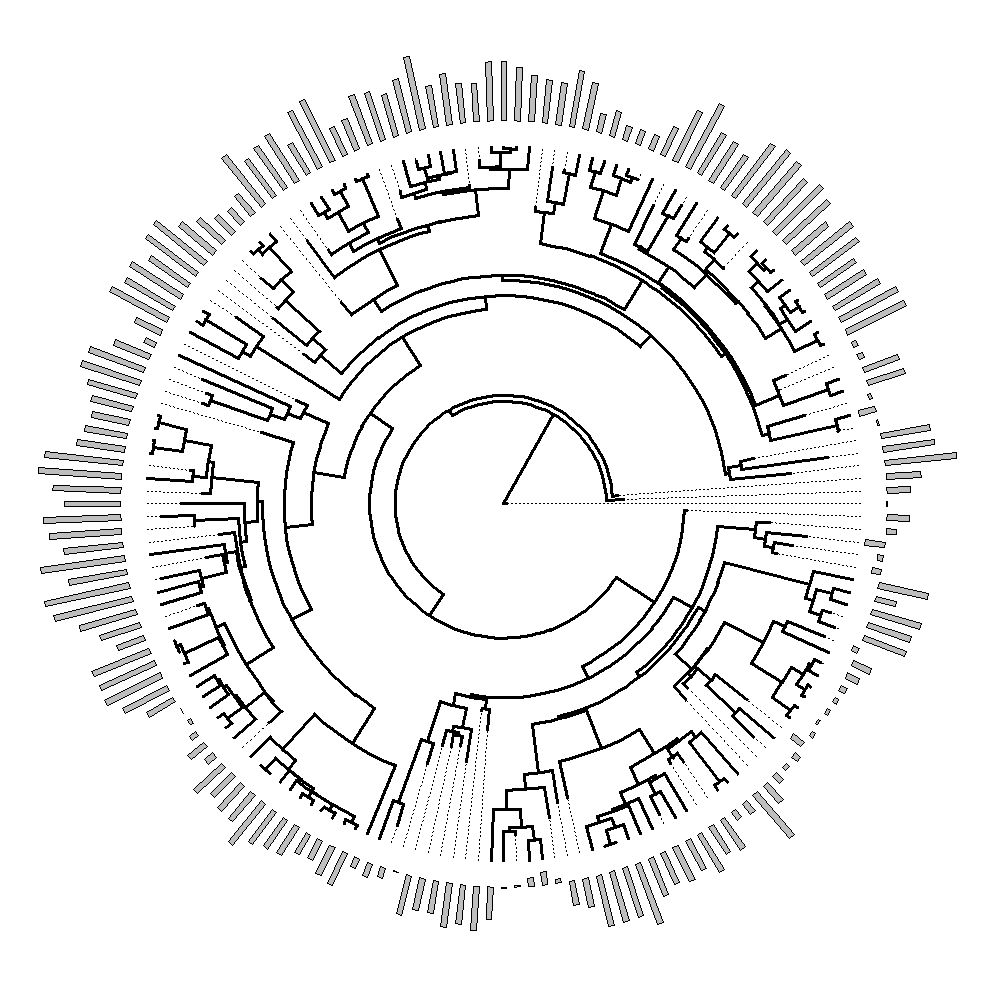Note that the default scale is different. The old version used an ignorant scale which could result in some pretty ludicrous plots by default. This should be better.